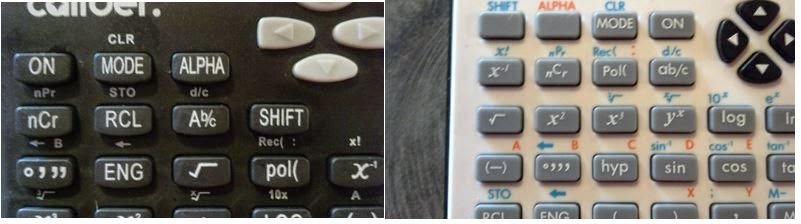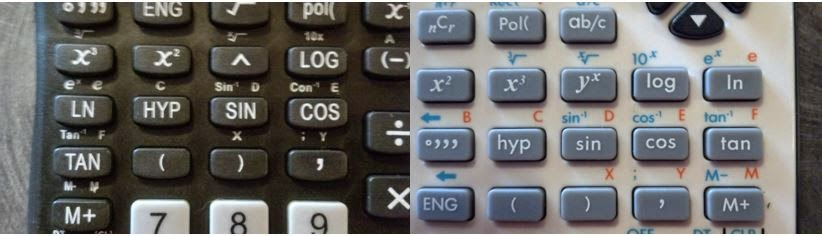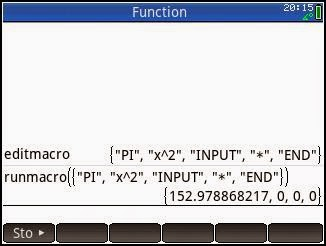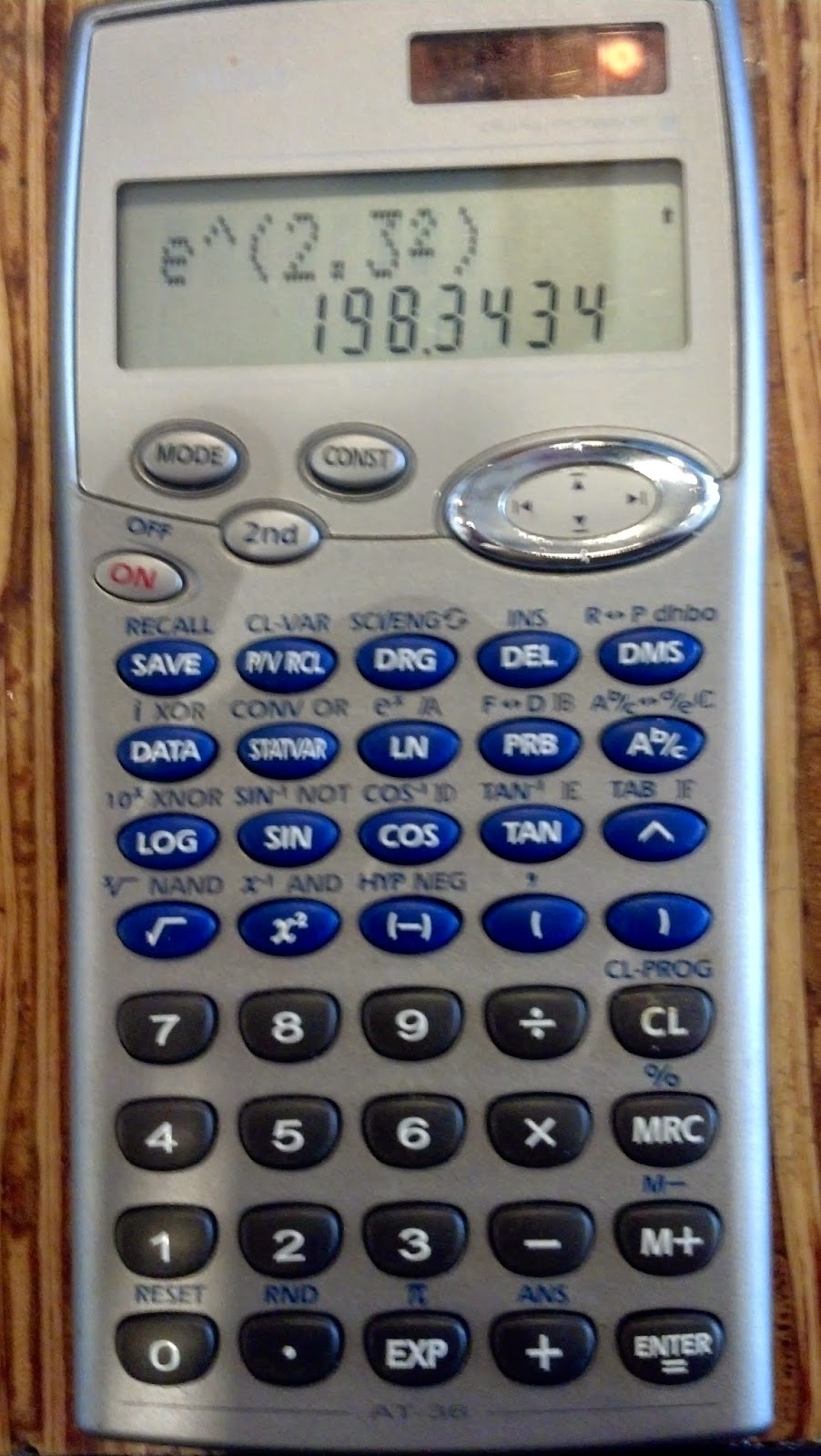## Tuesday, March 24, 2015

### A Ruler Calculator, Upcoming Equation Library Program for HP Prime, Calculator vs. Apps

Ruler Calculator

Shout out to Fischer Scientific!  My work had an office supplies fair yesterday.  It is not everyday that I see a ruler calculator.  I asked about how much is the ruler calculator because I wanted to order one for myself, and the nice vendor gave the ruler.  I am much appreciative!

Upcoming Equation Library Program for HP Prime

I am working an equation library program for the HP Prime, and so far I have 35 equations, and planning entering a few more.  Subjects covered include astronomy, finance, motion physics, sound, electronics, and Great Circle calculations.  I plan to release the program sometime in early April 2015 at the latest.

Calculator Apps versus Calculators (with tangible keyboards)

With the growing use of tables and smart phones, many calculator apps are available on Apple iOS devices (iPod Touch, iPad, iPhone) and Android devices.  Let me ask the readers: are you using calculator apps, and what is your opinion on them?  Do you have a preference between calculator apps or calculators?

Personally, I prefer calculators that have physical keyboards.  I like the tactile feel of the keys and the fact that calculators are single purpose machines.  It also frees my iPod for other tasks.  Having said that, two of my favorite calculator apps are CalcFxC and PCalc.  Calculator apps are also an excellent source of providing emulators of classic calculators.

Readers, I wish you the best, and have a great day!  Thank you for following, visiting, and commenting.

Eddie

This blog is property of Edward Shore.   2015.

## Saturday, March 21, 2015

### Caliber Scientific Calculator by CVS Pharmacy vs. Hewlett Packard HP 10s+

Caliber Scientific Calculator by CVS Pharmacy vs. Hewlett Packard HP 10s+

Last Friday, I just couldn't resist.  I was at CVS in Glendora, CA and purchased their Caliber Scientific Calculator, pretty much for its keyboard.CVS Caliber Scientific Calculator
Keyboard

On the surface, the Caliber Scientific Calculator is a basic level scientific calculator.  Notice the keyboard and its unique arrangement.  To see how it compares, let’s compare the keyboards of Caliber Scientific Calculator to the Hewlett Packard 10s+ Scientific Calculator.  They both share the same operating system, more on that later.HP 10s+ Scientific Calculator

Shift and Alpha MarkingsShift and Alpha markings (Caliber on the left, HP 10s+ on the right)
On the Caliber (on the left), unlike almost every single scientific calculator, the markings on the calculator, outside of the number keys, are all one color: white.  The primary functions are in white, the shift functions, white, and alpha characters, white.  The HP 10s+ (on the right) have different colors.  Shift functions are in sky blue and Alpha characters are in orange.

Placement of FunctionsTrigonometry Key Placement (Caliber on the left, HP 10s+ on the right)

Trigonometry Keys (pictured above):

Caliber places the [ SIN ] and [ COS ] on one row while the [ TAN ] key is on the next row.  Also noticed, the marking of the arccosine function:  Con⁻¹ instead of cos⁻¹.  Like every other calculator, the HP 10s+ places the three trigonometric keys in a row.

Alpha Characters:

Scientific calculators usually line up their alpha characters in linear and logical fashion.  Caliber defies this:

The B character (alpha of  [ ° ‘ “ ]) is placed one row above (!) the A character (alpha of [(-)]).  C, D, E, are on the next row (alpha of [HYP], [SIN], and [COS], respectively), and the F character (alpha of [TAN]) is on the next row.

Apparently, CVS took the keys of the HP 10s+, or most likely the Casio fx-300MSPlus, and rearranged the keys with not much regard to the placement or logic of the keys.

Operating System

Both the Caliber and HP 10s+ are clones of the Casio fx-300MSPLUS.  The three calculators have the following features:

* Fraction entry and simplification
* Nine memory registers (A through F, X, Y, M).  Storage addition and subtraction available for the variable M.
* Random Numbers
* Six statistic regression models:  linear, logarithmic, exponential, power, inverse, and quadratic.

General information for the fx-300MSPLUS:

Manual:

Verdict on the Caliber Calculator

Despite the unusual placement on the keys and the fact all the markings are one color, the Caliber operates well and expected.

Eddie

This blog is property of Edward Shore.  2015

### Regular Polygons: Internal Angles and Area

Find the Total Amount of Interior Angles

Start with a rectangular polygon where each side of length s.  This referrers to polygons with n sides.  (The pictures show a regular pentagon, where n = 5).   Draw a line from each vertex (corner) to the center of the polygon.  Note that n triangles are formed.  Label each internal angle as θ.

Note that each triangle has 180⁰ in angles.  Two of the angles of each triangle have measure half of internal angles (θ/2), and the third form a central angle.  Note that the sum of all the angles formed by the n triangles are 180⁰ * n, and:

180⁰ * n  = all interior angles + all central angles

The total of all central angle is 360⁰.  Hence:

180⁰ * n = all interior angles + 360⁰

(I)  all interior angles = 180⁰ * n - 360⁰

To find the angle of each interior angle, divide (I) by n:

(II)   each interior angle = θ = 180⁰ - 360⁰/n

Area of a Regular Polygon

Take one of the n triangles.  Determine by the height h by

tan(θ/2) = h/(s/2)
(III)  h = (s/2) * tan(θ/2)

And the area of each triangle is:

area = 1/2 * base * height
area = 1/2 * s * (s/2) * tan(θ/2)
(IV)   area = 1/4 * s^2 * tan(θ/2)

Taking each of the n triangles are into account, the total area (A) of regular polygon is:

A = n * area
(V)  A = n/4 *s^2 * tan(θ/2)

Note, we can state the area of the regular polygon in a separate form.

Substitute (II) into (V):

θ = 180⁰ - 360⁰/n
A = n/4 *s^2 * tan(1/2 *(180⁰ - 360⁰/n))
A = n/4 * s^2 * tan(90⁰ - 180⁰/n)

By the trigonometric identity cot(x) = tan(90⁰ - x) (see picture above),

(VI)  A = n/4 * s^2 * cot(180⁰/n)

To summarize, for a regular polygon:

Total of the interior angles:  180⁰ * n - 360⁰
Each interior angle:  θ = 180⁰ - 360⁰/n
Area of the regular polygon: A = n/4 *s^2 * tan(θ/2) = n/4 * s^2 * cot(180⁰/n)

This blog is property of Edward Shore.  2015

## Thursday, March 19, 2015

### HP Prime and Casio Prizm: Solar Irradiance

The program IRRAD will calculate two properties:

(1)  The solar angle of incidence given the angular elevation and azimuth (from south going “counterclockwise”:  east-north-west) of both the sun and panel.
(2)  The irradiance given by the solar panel.

Formulas

Angle of Incidence Given Azimuth

Degree mode is assumed, the angle of incidence (θ) is found in the following equation:

cos θ = cos(ep)*sin(es) + sin(ep)*cos(es)*cos(as-ap)

Where:
θ = angle of incidence
es = elevation of the sun
ep = elevation of the panel
as = azimuth of the sun, from south headed towards east, then north, then west

ap = azimuth of the panel, from south headed towards east, then north, then westAngle of IncidenceAzimuth and Elevation of the Sun and Solar Panel
Calculating Flux Density of Solar Radiation on a Surface

Calculating the flux density (energy) of solar radiation on a surface, Lambert’s Cosine Law is used.  Lambert’s Cosine Law states that the relation between the irradiance of the sun and the angle of incidence. The result is the irradiance of the surface.  Irradiance is the rate of energy (power) over a unit area.  In SI units, irradiance is measured in Watts per square meter (W/m^2).

ls = lb * cos θ

Where:
lb = the sun’s power or irradiance.  Often this is treated as a constant, which is approximately 1367 W/m^2 for extraterrestrial solar power, or approximately 1000 W/m^2 when we are dealing with the Earth’s surface (taking scattering of light into account)
ls = the panel’s power or irradiance

Deg
“SUN: ELEL, AZI(S)”
?->A:?->B
“PANEL: ELEV, AZI(S)”
?->C:?->D
“(W÷M²)”
cos C * sin A + cos A * sin C * cos(B-D)
cos¹ Ans -> θ
I * cos θ -> S
“INCIDENCE ANGLE”
θ
S

BEGIN
LOCAL es,as,ep,ap,θinc;
LOCAL ib,is;
HAngle:=1;
INPUT({es,as},”Sun”,{“Elev.:”,”Azi (S):”});
INPUT({ep,ap},”Panel”,{“Elev.:”,”Azi (S):”});
// angle of incidence
θinc:=ACOS(COS(ep)*SIN(es)+SIN(ep)*COS(es)*COS(as-ap));
MSGBOX(“Incidence Angle = “+θinc);
// Lambert’s Cosine Law
Is:=ib*COS(θinc);
RETURN {θinc,is};
END;

Example

Data:

Sun:
Elevation:  55⁰24’21” ≈ 55.40583⁰
Azimuth:  175⁰15’44” ≈ 175.26222⁰

Panel:
Elevation:  40⁰
Azimuth:  90⁰ (panel is facing due east)

Irradiance of the Sun:  1000 W/m^2

Output:
Incidence Angle ≈ 48.643169⁰

Sources:

Baldocchi, Dennis “Lecture 7, Solar Radiation, Part 3, Earth-Sun Geometry”  Biometeorogy, ESPM 129  University of California, Berkeley.
Retrieved February 17, 2015.

Mortimer, David  “Lambert’s Cosine Law”  30 January 2014.  The Solar Bucket.
Retrieved March 18, 2015

University of Oregon Solar Radiation Monitoring Laboratory  “Solar Radiation Basics”  University of Oregon.  http://solardat.uoregon.edu/SolarRadiationBasics.html   Retrieved February 10, 2015

This blog is property of Edward Shore – 2015.

## Tuesday, March 17, 2015

### Quilts: Finding the Number of Blocks That Fit in a Rectangle

Quilts:  Finding the Number of Blocks That Fit in a Rectangle

The program QUILT will find the number of small tiles that will fit in a large rectangular quilt (this can be applied to game boards, posters, or other decorative items).  QUILT will also calculate the number of columns and rows of tiles you will have, and the leftover area.  The idea is to minimize the area that remains after placing the maximum amount of tiles.  I will assume that no tiles will be cut in the process.Fitting Tiles to a Quilt
Where:
bw = width of the quilt (labeled A on the Prizm program QUILT)
bl = length of the quilt (labeled B on the Prizm program QUILT)
sw = width of the tile (labeled C on the Prizm program QUILT)
sl = length of the tile (labeled D on the Prizm program QUILT)

Formulas

Number of tiles that can fit:
n = floor(bw/sw) * floor(bl/sl) = int(bw/sw) * int(bl/sl)
where floor is the floor function and int represents the integer function.  Essentially, you want the integer (whole number) portion of the divisions sw/sl and bw/bl and disregard the fraction part.

Number of columns of tiles = floor(bw/bl)

Number of rows of tiles = floor(sw/sl)

Leftover area = bw * bl – n * sw * sl

Thanks to

Casio Prizm: QUILT

“QUILT WIDTH AND”
“LENGTH:”
? -> A
? -> B
“TILE WIDTH AND”
“LENGTH:”
? -> C
? -> D
Int (A÷C) * Int (B÷D) -> N
AB – NCD -> L
“NUMBER OF TILES”
N
“COLUMNS=”
Int (A÷C)
“ROWS=”
Int (B÷D)
“AREA LEFTOVER=”
L

HP Prime:  quilt

quilt()
BEGIN
LOCAL bw, bl, sw, sl, n, l;
INPUT({bw,bl},”Quilt”,{“Width:”, “Length:”});
INPUT({sw,sl},”Tile”,{“Width:”, “Length:”});
n:=FLOOR(bw/sw)*FLOOR(bl/sw);
l:=bw*bl-sw*sl*n;
PRINT()
PRINT(“# of tiles = “+ n);
PRINT(“# of columns = “+FLOOR(bw/sw));
PRINT(“# of rows = “+FLOOR(bl/sl));
PRINT(“Leftover Area = “+l);
RETURN {n,FLOOR(bw/sw),FLOOR(bl/sl),l};
END;

Examples

Example 1:
We have a quilt that measures 40” by 54” and we want to fit as many tiles measuring 2” by 3”.  Hence:  bw = 40, bl = 54, sw = 2, and sl = 3.

Result:
Number of tiles:  360
Number of columns: 20
Number of rows: 18
Leftover area:  0  (perfect fit!)

Example 2:
We have a quilt that measures 48” by 54” and we want to fit as many tiles measuring 5” by 5”.  Hence:  bw = 48, bl = 54, sw = 5, and sl = 5.

Result:
Number of tiles:  90
Number of columns: 9
Number of rows: 10
Leftover area:  342 sq inches  (perfect fit!)

Happy St. Patrick’s Day!

Eddie

This blog is property of Edward Shore.  2015

## Saturday, March 14, 2015

### Happy Pi Day!

Happy Pi Day!  50 Digits of Pi

https://youtu.be/BVzVeFzz1eU

Eddie

This blog is property of Edward Shore. 2015

## Wednesday, March 11, 2015

### Some of My Favorite Websites to Visit

Here are some of my favorite mathematical websites to visit.

Museum of HP Calculators (MoHPC)

This site contains a history of Hewlett Packard calculators and has a forum for calculator owners and mathematical enthusiasts.  The forum contains a software library for many of the HP programmable calculators, with threads dedicated to the HP 41C, HP 67, and the HP Prime.  The people on this forum are incredibly smart and very friendly.

Wolfram Alpha

This is a dream website.  Here you can type almost any question regarding math, chemistry, physics, biology, and astronomy; and get straight forward answers about the subjects that you desire.   I often ask Wolfram Alpha for astronomical locations of planets, our sun, and our constellations.

Wolfram Alpha also has a CAS engine which will compute indefinite integrals, expand polynomials, produce truth tables, factorize integrals, and much more.   Wolfram Alpha is also has apps available on iOS and Android for \$2.99 - well worth the price.

Cemetech

The web site, run by Christopher R. Mitchell, the author of "Using the TI-83 Plus/TI-84 Plus" (a super book).  This website has technical news and forum for members.  I just recently joined the forum.  Cemetech primarily focuses on Texas Instruments calculators, but there is also a program archive for the Casio Prizm and the HP Prime. There are many developers that share their programs and user-made applications for the TI calculators on Cemetech.

Datamath

This website in is an online album of ever Texas Instruments calculators ever produced since the 1970s.  You can down manuals to classical TI calculators including a library for the TI-58/59 calculator.

RSKEY.ORG

This site is an online album of any programming and graphing calculators of all the companies, not just the big three (TI, HP, Casio).  I can easily spend a few hours on this website.

hpcalc.org

If you need a place to find good programs for the HP 48, HP 28, HP 49, HP 50g, HP 39, and HP Prime, this is a good place to go.  This website is hosted by Eric Rechlin.

Tech Powered Math

This is an educational website that covers math and graphing calculators.  The website is run by Lucas Allen, author of “TI-Nspire Tutorials: The TI-nSpire for Beginners”.

And a website that has nothing to do with calculators but with movies...

CinemaSins

This is the official CinemaSins page, you may have seen the more familiar YouTube channel.  The CinemaSins counts all the quirks, mistakes, and funny coincidences of popular movies.  Jeremy Scott is the phenomenal narrator.  CinemaSins have expanded to count the sins of popular brands and music videos.

So that is some of my favorite places to visit.  If these websites are new to you, I hope you check them out and enjoy them.

Have a great day everyone!  Eddie

This blog is property of Edward Shore.  2015

## Sunday, March 8, 2015

### HP Prime: RPN Keystroke Programming Simulatoreditmacro and runmacroHelps Screen on editmacro

Bring RPN keystroke programming to the HP Prime with editmacro and runmacro.

Build the program with editmacro.  The result is a list of strings which make the macro.  Save the string to any variable you want.  Use runmacro to execute the string of commands.

Features:

*  Standard Scientific Functions
*  Polar and Rectangular conversions
*  Hyperbolic Functions
*  Standard Normal Distribution Lower Tail and Inverse Functions
*  Complex Number Functions: Real, Imaginary, Absolute Value, and Argument
*  20 temporary register variables which can be used within each macro.  Storage arithmetic, increment and decrement by 1 are included.
*  9 labels - subroutines one level deep
*  Six tests:  x>0?, x<0?, x=0?, x>y?, x<y?, x=y?.  They work in the classic RPN keystroke format.

Eddie

This blog is property of Edward Shore - 2015

## Saturday, March 7, 2015

### Datexx DS 834, Ativa AT-36, and Clones: The Mystery of the CL-PROG function

If you have a Datexx DS-834 solar calculator, or any of the clones, such as the Ativa AT-36 calculator (pictured below):Ativa AT-36 Calculator.  I bought from an Office Depot store before it was merged with Office Max eons ago.  Compared to today's standards, this calculator is slow as heck!

The calculator would feature a "CL-PROG" function as the second function of the Clear key, as shown below.The CL button, with the CL-PROG function

This would imply that the Datexx DS 834 ECO (Link:  http://www.datexx.com/product-p/ds-834.htm), Ativa AT-36, and any other clones, such as the Citizen SRP-285N (Link:  http://calculator.citizen-europe.com/en/calculator/srp-285n )  would be programmable.

However, looking over the five modes which are:

Main Mode: Calculator mode
Stat Mode:  Statistics mode (1 variable, 2 variable? )
Base-n Mode:  Boolean Algebra with decimal/binary/octal/hexadecimal
Complex Mode:  Arithmetic Only
VLE Mode:  2 x 2 Simultaneous Linear Equation Solver

No program or formula programming mode is available. No mention of the use of the CL-PROG feature in the any of the manuals or the example sheets provided.  I don't know if this feature was going to be programmed and the original programmers just ran out of time.  However, the [2nd] [ CL ] for CL-PROG seems to do nothing.  If you do know, please comment on this blog entry.

One of the curiosities of scientific calculators.

Eddie

This blog is property of Edward Shore - 2015

## Tuesday, March 3, 2015

### Casio Prizm and TI-84+: Modulus Program

Casio Prizm and TI-84+: Modulus Program

Formula:
A MOD B = B * frac(A/B) + B * (A<0 and B>0) + B * (B<0 and A>0)
(updated 1/6/2016)

Remove the prompt of A and B to make MODDIV suitable as a subroutine.

Casio Prizm: MODDIV (including fx-9860g & fx-9750g)
"A"? → A
"B" → B
B × Frac (A ÷ B) + B × (A < 0 And B > 0) + B × (B < 0 And A > 0)

TI-84: MODDIV
Prompt A, B
B * fPart(A/B) + B * (A<0 and B>0) + B * (B<0 and A>0)

Examples:

A mod B = R

A = 48, B = 3, R = 0
A = -52, B = 6, R = 2
A = 41.3, B = 12, R = 5.3
A = -50.2, B = 36, R = 21.8
A = 48, B = -7, R = -1

This blog is property of Edward Shore. 2015

### HP Prime: Solar Position (Right Ascension, Declination, Altitude, Azimuth)

HP Prime: Solar Position (Right Ascension, Declination, Altitude, Azimuth)

Input:
* Month
* Date
* Year
* Local Time (your local standard time – do not adjust for daylight savings time)
* Longitude
* Latitude

Local Time:  Use a 24 Hour clock. When entering time, you can enter a decimal or hours°minutes’seconds’’.

Longitude:  This is your location, going east from the Greenwich Prime Meridian.  East is positive, West is negative. Range: -180° to 180°

Latitude: This is your location, going north from the Equator.  North is positive, South is negative.  Range:  -90° to 90°

Entering HMS:

HP Prime:  Use Shift+9 and select the appropriate symbol (°, ‘, or ‘’)

Output:
r:  Distance from the Earth to the Sun in astronomical units (AU)
α:  Right ascension in decimal hours
δ:  Declination in decimal degrees
eot:  Equation of Time in minutes
alt:  Altitude/Elevation
azi:  Azimuth, from due North going clockwise

Also, a 2-column matrix is returned to the home screen for reference.  The first column is the input column, the second is the output column.

[[ month,  distance ]
[ day,  right ascension ]
[ year, declination ]
[ local time, declination ]
[ longitude, altitude ]
[ latitude, azimuth ]]

HP Prime:  solar

EXPORT solar()
BEGIN
// aa.usno.navy.mil
// Updated 2015-03-01 EWS

// month, day, year, local standard
// time, longitude, latitude
LOCAL m,D,Y,lstd,long,lat;

INPUT({m,D,Y,lstd,long,lat},
"Data: Use Shift+9 for H°M′S″",
{"Month:","Date :","Year :",
"Local Time (24):", "Long (+E):",
"Lat (+N)"});

// Initialization
LOCAL d,g,q,L,r,ec,gmt;
LOCAL eot,alt,lha,azi,zen,loc;
LOCAL α,δ,g1,g2;
LOCAL w1,w2;
HAngle:=1;

// Greenwich Mean Time
gmt:=lstd-long/15;

// Julian Date
d:=367*Y-IP(7*(Y+IP((m+9)/12))/4)
+IP((275*m)/9)+D+1721013.5+gmt/24
-0.5*SIGN(100*Y+m-190002.5)+0.5;
d:=d-2451545;

// Intermediate Calculations
g:=(357.529+.98560028*d)  MOD 360;
q:=(280.459+.98564736*d) MOD 360;
L:=(q+1.915*SIN(g)+.02*SIN(2*g)) MOD 360;
r:=1.00014-.01671*COS(g)-.00014*COS(2*g);
ec:=23.439291-.00000036*d;

α:=ARG(COS(L)+SIN(L)*COS(ec)*i) MOD 360;

// Convert to hours
α:=α/15;

// Declination
δ:=ASIN(SIN(ec)*SIN(L));

// Equation of Time
eot:=q/15-α;
eot:=eot*60;

// Greenwich Mean Time (in hours)
g1:=-0.000319*SIN(−125.04-0.052954*d)
-2.4ᴇ−5*SIN(560.94+1.9713*d);
gmt:=(18.697374558+24.06570982441908*d
+g1*COS(ec)) MOD 24;

// Hour Angle (in degrees)
lha:=(gmt-α)*15+long;

// Altitude (approximate)
alt:=ASIN(SIN(lat)*SIN(δ)+
COS(lat)*COS(δ)*COS(lha));

// Azimuth
// Clockwise from South
azi:=ARG(SIN(lha)*i+COS(lha)*SIN(lat)
-TAN(δ)*COS(lat));
// Convert to clockwise from North
azi:=azi+180;

PRINT();
PRINT("Sun "+m+"/"+D+"/"+Y+" ; "+lstd);
PRINT("Distance: "+r);
PRINT("α (hours): "+α);
PRINT("δ (degrees): "+δ);
PRINT("eot (minutes): "+eot);
PRINT("Approximate");
PRINT("alt (elevation): "+alt);
PRINT("azi (North-clockwise): "+azi);

PRINT("DEGREES MODE SET");
RETURN [[m,r],[D,α],[Y,δ],[lstd,eot],
[long,alt],[lat,azi]];
END;

Example:

Input:
June 1, 2015; 12:00 PM , Longitude: -118°13’59”, Latitude: 34°3’

Output:
r = 1.01406353128 AU
α = 4.6292012064 hr
δ = 22.0919016903°
eot = 2.157339456 min
alt = 78.032250726°
azi = 182.442424012°

Resources:

The United States Naval Observatory (USNO)  Washington, D.C.:
“Approximate Solar Coordinates” (URL:  http://aa.usno.navy.mil/faq/docs/SunApprox.php )
“Approximate Sidereal Time” (URL: http://aa.usno.navy.mil/faq/docs/GAST.php )
“Computing Altitude and Azimuth from Greenwich Apparent Sidereal Time” (URL: http://aa.usno.navy.mil/faq/docs/Alt_Az.php )
“Converting Between Julian Dates and Gregorian Calendar Dates” (URL: http://aa.usno.navy.mil/faq/docs/JD_Formula.php )
Retrieved February 23, 2015 to March 1, 2015

This blog is property of Edward Shore - 2015

## Sunday, March 1, 2015

### HP Prime & TI-84+: Bolt Pattern

HP Prime & TI-84+:  Bolt PatternBolt Pattern

The program BOLTPAT will calculate a bolt pattern given:
X = center, X coordinate
Y = center, Y coordinate
N = number of bolts
D = diameter of the circle

No angle of rotation is assumed.

Coordinates of each bolt can be calculated by:

X_k = (d/2*COS(360*k/n)+xc)
Y_k = (d/2*SIN(360*k/n)+yc)

Where k = 0 to n-1

The OC-Distance between each bolt is calculated by calculating the linear distance between neighboring bolts.

Output:
Each point where the bolt should be placed.  In addition, the on-center distance between bolts is displayed.  Finally, each of the coordinates will be presented in a list.  For the TI-84+ version, the list will be stored in list L6.

HP Prime:  BOLTPAT

EXPORT BOLTPAT()
BEGIN
// EWS 2012-02-28
// No pre angle tilt

// Degrees
HAngle:=1;

// Data
LOCAL z0,z1,xc,yc;
LOCAL z,n,d,k,l,xp,yp;
INPUT({xc,yc,n,d},"Bolt Spacing",
{"xc:","yc:","n :","d :"},
{"x center","y center",
"number of bolts",
"diameter"});
l:={};

// Calculation
PRINT();
PRINT("x + y*i");
FOR k FROM 0 TO n-1 DO
xp:=(d/2*COS(360*k/n)+xc);
yp:=(d/2*SIN(360*k/n)+yc);
IF k==0 THEN
z0:=xp+i*yp;
END;
IF k==1 THEN
z1:=xp+i*yp;
END;
l:=CONCAT(l,{xp+yp*i});
PRINT("("+xp+","+yp+")");
END;
PRINT("OC-OC: "+ABS(z1-z0));

RETURN l;

END;

TI-84+: BOLTPAT

a+bi
Degree
Disp “X: X CENTER”
Disp “Y: Y CENTER”
Disp “N: NO. BOLTS”
Disp “D: DIAMETER”
Prompt X,Y,N,D
For(K,0,N-1)
D/2*cos(360*K/N)+X→S
D/2*sin(360*K/N)+Y→T
If K=0
Then
S+T*i→U
{U}→L6
End
If K>0
Then
Augment(L6,{S+T*i})→L6
End
If K=1
Then
S+T*i→V
End
Disp “POINT”,K
Disp S
Disp T
Pause
End
Disp “OC-OC:”
Pause abs(U-V)
Disp L6

Example:

Build a bolt pattern with 5 bolts of a circle of diameter of 3 units.  The center of the circle has the center (4, 1).  (X = 4, Y = 1, N = 5, D = 3)

Bolts should be placed at the following points:
(5.5, 1)
(4.4635, 2.4266)
(2.7865, 1.8817)
(2.7865, 0.1183)
(4.4635, -0.4266)

OC-Distance:  1.7634

Note:  Bolt Patterns can easily be calculated with the Machinist Calc Pro, original (Model 4087) or Pro 2 (Model 4088) (including angle of rotation).

This blog is property of Edward Shore – 2015.

### TI-Nspire: Templates to Plot Functions, Parametric Equations, and Sequences

TI-Nspire:  Templates to Plot Functions, Parametric Equations, and Sequences Introduction The following are sample templates to plot functi...